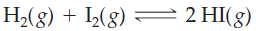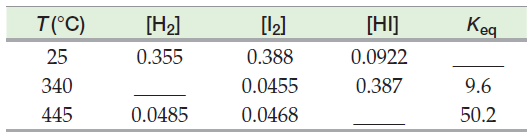×
Get Full Access to Introductory Chemistry - 5 Edition - Chapter 15 - Problem 62p
Get Full Access to Introductory Chemistry - 5 Edition - Chapter 15 - Problem 62p

×

# Consider the reaction: Complete the table. Assume that all concentrations areISBN: 9780321910295 34

## Solution for problem 62P Chapter 15

Introductory Chemistry | 5th Edition

• Textbook Solutions
• 2901 Step-by-step solutions solved by professors and subject experts
• Get 24/7 help from StudySoup virtual teaching assistantsIntroductory Chemistry | 5th Edition

4 5 1 270 Reviews
14
0
Problem 62P

Consider the reaction:Complete the table. Assume that all concentrations are equilibrium concentrations in moles per liter, M.Step-by-Step Solution:

Solution 62P :

Step 1:

Given the reaction:

H2(g) + I2(g) ⇋ 2HI(g)

Here, we have to complete the table assuming that all concentrations are equilibrium concentrations in moles per liter, M.

 T(°C) H2 [I2] [HI] Keq 25 0.355 0.388 0.0922 ______ 340 ______ 0.0455 0.387 9.6 445 0.0485 0.0468 ______ 50.2

We know the formula for equilibrium constant (Kc or Keq):

Keq =, i.e  Keq =Now, consider 1st row :

T(°C) = 25

H2 = 0.355

[I2] = 0.388

[HI] = 0.0922

Keq = ?

Hence, Keq == 0.0617

Step 2 of 4

Step 3 of 4

##### ISBN: 9780321910295

Introductory Chemistry was written by and is associated to the ISBN: 9780321910295. This full solution covers the following key subjects: concentrations, Equilibrium, assume, consider, complete. This expansive textbook survival guide covers 19 chapters, and 2046 solutions. The answer to “?Consider the reaction: Complete the table. Assume that all concentrations are equilibrium concentrations in moles per liter, M.” is broken down into a number of easy to follow steps, and 18 words. Since the solution to 62P from 15 chapter was answered, more than 412 students have viewed the full step-by-step answer. This textbook survival guide was created for the textbook: Introductory Chemistry, edition: 5. The full step-by-step solution to problem: 62P from chapter: 15 was answered by , our top Chemistry solution expert on 05/06/17, 06:45PM.

Unlock Textbook Solution